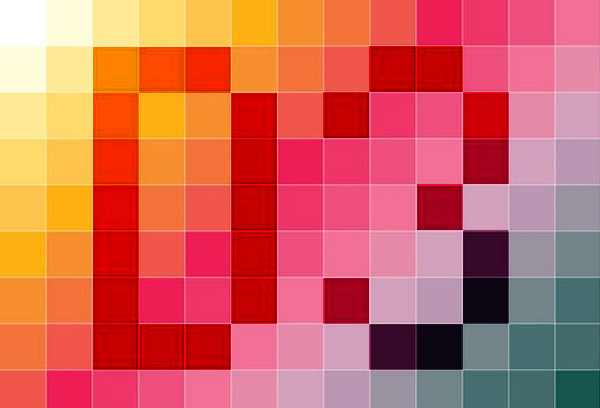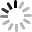# SVG D3.js - 定義色彩 - 基本篇 ( colors )• d3.rgb
• rgb.brighter
• rgb.darker
• rgb.hsl
• rgb.toString

## d3.rgb(r, g, b)

HTML：

``````<div id="red"></div>
<div id="green"></div>
<div id="yellow"></div>
<div id="blue"></div>
``````

JS：

``````var d = d3.selectAll('div'),
r = d3.select('#red'),
g = d3.select('#green'),
y = d3.select('#yellow'),
b = d3.select('#blue');

d.style({
'width':'80px',
'height':'80px',
'display':'inline-block',
'margin':'3px'
});

var red = d3.rgb(255,0,0);
var green = d3.rgb(0,255,0);
var yellow = d3.rgb(255,255,0);
var blue = d3.rgb(0,0,255);

r.style({
'background':red
});
g.style({
'background':green
});
y.style({
'background':yellow
});
b.style({
'background':blue
});
``````## d3.rgb(color)

``````var red = d3.rgb('red');
var green = d3.rgb('green');
var yellow = d3.rgb('yellow');
var blue = d3.rgb('blue');
````````````var red = d3.rgb('#f00');
var green = d3.rgb('#0f0');
var yellow = d3.rgb('#ff0');
var blue = d3.rgb('#00f');
``````## rgb.brighter([k])

``````var red = d3.rgb(50,0,0);

r.style({
'background':red.brighter(0)
});
r1.style({
'background':red.brighter(1)
});
r2.style({
'background':red.brighter(2)
});
r3.style({
'background':red.brighter(3)
});
r4.style({
'background':red.brighter(4)
});
````````````brighter: function (n){n=Math.pow(.7,arguments.length?n:1);var t=this.r,e=this.g,r=this.b,u=30;return t||e||r?(t&&u>t&&(t=u),e&&u>e&&(e=u),r&&u>r&&(r=u),new dt(Math.min(255,t/n),Math.min(255,e/n),Math.min(255,r/n))):new dt(u,u,u)}
``````

## rgb.darker([k])

``````var red = d3.rgb(255,0,0);

r.style({
'background':red.darker(0)
});
r1.style({
'background':red.darker(1)
});
r2.style({
'background':red.darker(2)
});
r3.style({
'background':red.darker(3)
});
r4.style({
'background':red.darker(4)
});
``````## rgb.hsl()

``````var red = d3.rgb(255,0,0);
console.log(red.hsl());
``````## rgb.toString()

``````var red = d3.rgb(255,0,0);
console.log(red.toString());
``````